# Permutation group

The group consisting of all possible permutations of N objects is known as the permutation group or symmetric group. A permutation is an invertible function from a set to itself. The group is usually denoted by SN. It is a group of order N! (N factorial) and is a central object of study in group theory.

## Permutations

Consider a linear array A of N objects, numbering the positions in the array from 1 to N. A permutation π, designated as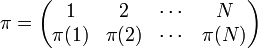$\pi = \begin{pmatrix}1 & 2 & \cdots & N \\ \pi(1) & \pi(2) & \cdots & \pi(N) \\ \end{pmatrix}$

is the following map: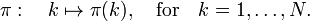$\pi:\quad k \mapsto \pi(k), \quad\hbox{for}\quad k=1, \ldots, N.$

That is, π moves the object in position k of the linear array A into position π(k); this is the active convention, the objects are moved as the pieces on a chessboard.

This map is surjective (onto) by definition as well as injective, i.e. k ≠ l implies that π(k) ≠ π(l). A permutation can thus be defined as a one-to-one map (bijection) of {1, 2, ..., N} onto itself. Since it is assumed that the positions in the array A are counted from left to right, there is no need to number the positions of A explicitly. Furthermore, A is loosely referred to as a "set" of objects with elements written between braces. The set obtained from A by the action of π is denoted by π(A).

Example\begin{align} A &= \{\,E,\,\;P,\,\;J,\,\;P,\,\;J,\,\;W,\;P,\;W\} \\ \pi &= \begin{pmatrix}1 & 2 & 3 & 4 & 5 & 6 & 7 & 8 \\ 3 & 8 & 7 & 2 & 1 & 6 & 5 & 4 \\ \end{pmatrix} \\ \pi(A)&= \{\,J,\,\; P,\,\; E,\,\; W,\,\; P,\,\; W,\; J,\; P\}. \\ \end{align}

For a set where the objects are numbered, e.g.,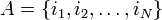$A = \{i_1, i_2, \ldots, i_N\}$, the effect of π on the subscripts (the numbering) is$\pi(A) = \{i_{\pi^{-1}(1)}, i_{\pi^{-1}(2)}, \ldots, i_{\pi^{-1}(N)}\}.$

In order to follow consistently the active convention, the inverse π−1 of π appears here. The inverse of π is the permutation that undoes the effect of π (see below). An alternative, yet valid, convention of defining the permutation π is the following (passive) one: an object in position k is replaced by an object in position π(k), for k= 1, ..., N successively. In the example above this would yield the set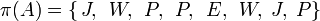$\pi(A)= \{\,J,\,\; W,\,\; P,\,\; P,\,\; E,\,\; W,\; J,\; P\}$

or, generally, the passive convention is,$\pi: \quad\{i_1, i_2, \ldots, i_N\} \rightarrow \{i_{\pi(1)},i_{\pi(2)},\ldots, i_{\pi(N)}\}.$

This interpretation clearly gives permutations that are the inverse of the active convention. It is obvious that one must adhere to one chosen convention.

Application of π to A followed by application π′ defines a new permutation π′′, the product—or composition—of π and π′. This is written as π′′ = π′ π.

Example$\begin{pmatrix} 1&2&3&4&5 \\ 1&5&2&3&4 \\ \end{pmatrix} = \begin{pmatrix} 1&2&3&4&5 \\ 4&3&1&2&5 \\ \end{pmatrix} \begin{pmatrix} 1&2&3&4&5 \\ 3&5&4&2&1 \\ \end{pmatrix}.$

(The rightmost permutation π moves the object in position 1 to position 3, then second permutation π' moves the object in position 3 to position 1. The object in position 2 goes to position 5 and then stays there, etc.).

It follows immediately from this definition that the multiplication of permutations is associative: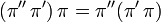$(\pi''\,\pi')\,\pi = \pi''(\pi'\,\pi)$.

Furthermore, a permutation π, being a one-to-one map of {1, 2, ..., N} onto itself, has a unique inverse, denoted by π−1 , with the property$\pi\, \pi^{-1} = \pi^{-1}\, \pi = e.$

The identity element e is the identity permutation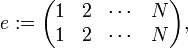$e: = \begin{pmatrix} 1 & 2 & \cdots & N \\ 1 & 2 & \cdots & N \\ \end{pmatrix},$

which is the map sending each element to itself. The inverse permutation π−1 is simply obtained by interchanging the two rows of π (i.e., interchange of the domain and the range of π).

Example$\pi = \begin{pmatrix} 1&2&3&4 \\ 4&3&2&1 \\ \end{pmatrix} \quad \hbox{gives} \quad \pi^{-1} = \begin{pmatrix} 4&3&2&1 \\ 1&2&3&4 \\ \end{pmatrix} = \begin{pmatrix} 1&2&3&4 \\ 4&3&2&1 \\ \end{pmatrix}$

## Permutation group

The associativity of the product, the existence of an identity permutation and the existence of a unique inverse of a permutation imply that the set of all permutations forms a group. This is the permutation (or symmetric) group, commonly denoted by SN. Since there are N! different one-to-one maps of {1,2,..., N} onto itself, this group has the order N! (the factorial function).

## Cycle notation

Often a more concise notation for permutations is used. Define a cycle c of length λ (a λ-cycle), denoted by$c = (i_1, i_2, i_3, \ldots, i_\lambda),$

as the permutation πc$\pi_c \equiv c := \begin{pmatrix} i_1 & i_2 & i_3 &\cdots & i_{\lambda-1} & i_\lambda \\ i_2 & i_3 & i_4 & \cdots &i_\lambda &i_1 \\ \end{pmatrix},$

which moves an object in position i1 to position i2 an object in position i2 to position i3, etc., until finally an object in position iλ to position i1.

Example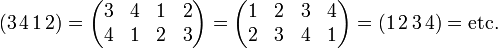$(3\,4\,1\,2) = \begin{pmatrix} 3&4&1&2 \\ 4&1&2&3 \\ \end{pmatrix}= \begin{pmatrix} 1&2&3&4 \\ 2&3&4&1 \\ \end{pmatrix} = (1\,2\,3\,4) = \hbox{etc.}$

Any permutation can be written as a product of disjoint cycles.

Example\begin{align} \pi &= \begin{pmatrix} 1&2&3&4&5&6&7&8 \\ 2&3&1&6&7&5&4&8 \\ \end{pmatrix} = (1\,2\,3)(4\,6\,5\,7)(8) \\ \pi^{-1} &= \begin{pmatrix} 1&2&3&4&5&6&7&8 \\ 3&1&2&7&6&4&5&8 \\ \end{pmatrix} = (3\,2\,1)(7\,5\,6\,4)(8). \end{align}

This example also illustrates the fact that the inverse of$(i_1, i_2, i_3, \ldots, i_\lambda)$ is$(i_\lambda, i_{\lambda-1}, i_{\lambda-2}, \ldots, i_1)$; simply reverse the order within the cycles, but the number and lengths of cycles is unchanged.

It is common to omit the 1-cycles from a permutation written in cycle notation, except in the case of identity permutation e = (1)(2) ⋅ ⋅ (N), which in cycle notation is usually denoted by (1). The element appearing in a 1-cycle is sometimes referred to as a fixed point of the permutation because it is not moved by the permutation.

The order of a permutation is the least common multiple of the cycle lengths in the cycle decomposition.

## Permutational parity

One defines the parity (also known as sign or signature) of a λ-cycle as the number (−1)λ+1. If this number is 1, the cycle has even parity, if it is −1, the cycle has odd parity. Thus, 1-cycles are of even parity, 2-cycles (transpositions) are of odd parity, and so on. If a permutation π is factorized into (not necessarily disjoint) cycles,$\pi = c_1\, c_2 \cdots c_k,$

where ci is of length λi, then the parity of π, denoted by (−1)π, is defined by the product of the parities of the constituting cycles,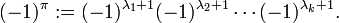$(-1)^\pi := (-1)^{\lambda_1+1} (-1)^{\lambda_2+1} \cdots (-1)^{\lambda_k+1}.$

For example, the permutation π = (1 3 2)(4 5) has the parity$(-1)^\pi = (-1)^4 (-1)^3 = -1.\;$

Clearly, π−1 has the same parity as π, since the cycle structure (the number and lengths of cycles) of π is the same as of π−1. Cycle structure is also known as cycle shape.

An alternative and completely equivalent way to define permutational parity is by decomposition of a permutation into 2-cycles (transpositions). A cycle can be written as a product of transpositions,$(a~b~\ldots y~z) = (a~z)(a~y)\cdots(a~b)$, and hence every permutation in SN, for N > 1, can be written as a product of transpositions. A permutation of N objects is then called even if it can be written as the product of an even number of transpositions and odd if it can be written as the product of an odd number of transpositions. The nontrivial fact about this terminology is that it is well-defined; that is, no permutation is both even and odd. Note that the number of transpositions factoring a permutation is not fixed, it is always possible to multiply by an even number of transpositions that give the identity element. For instance, a cycle c is equal to c(i j)(j i)(k ℓ)(ℓ k).

The even permutations in SN form a subgroup of SN. This subgroup is called the alternating group on N objects and denoted by AN. In fact, AN is always a normal subgroup of SN, all odd permutations being in a coset that is both a left and a right coset of AN. The order of AN is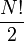$\frac{N!}{2}$.

## Conjugacy classes

From elementary group theory it is known that the conjugacy relation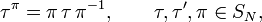$\tau^{\pi} = \pi\,\tau\,\pi^{-1}, \qquad \tau, \tau', \pi \in S_N,$

is an equivalence relation, i.e., the permutations τ and τπ belong to the same equivalence class, referred to as a conjugacy class.

There is a simple way to compute τπ from a given τ and π. Consider π: k → π(k), for k = 1, ..., N, successively, and let τ be written in cycle notation. Then the permutation τπ is obtained by replacing in τ the number k by the number π(k) for all k.

Example

For$\tau = (1\,3\,2)(4\,5)\quad \hbox{and} \quad \pi = \begin{pmatrix} 1&2&3&4&5&6 \\ 1&6&4&5&3&2 \\ \end{pmatrix}$

we have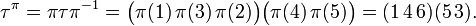$\tau^{\pi} = \pi\tau\pi^{-1} = \bigl(\pi(1)\,\pi(3)\,\pi(2)\bigr) \bigl(\pi(4)\,\pi(5)\bigr) = (1\,4\,6)(5\,3).$

One can easily verify this rule by computing π−1 and by doing the required multiplications. The rule immediately implies that any two conjugate permutations have the same cycle structure (cycle shape), i.e., they consist of the same number of cycles of the same length.

Conversely, if two permutations τ and τ′ have the same cycle structure, there exists at least one permutation π such that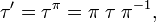$\tau' = \tau^{\pi} = \pi\;\tau\;\pi^{-1},$

so that equal cycle structure implies conjugacy. The permutation π can easily be found from a given pair τ and τ′ by inverting the recipe given above for the calculation of τπ.

Example

For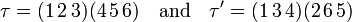$\tau = (1\,2\,3)(4\,5\,6) \quad \hbox{and} \quad \tau' = (1\,3\,4)(2\,6\,5)$

it follows that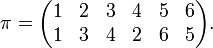$\pi = \begin{pmatrix} 1&2&3&4&5&6 \\ 1&3&4&2&6&5 \\ \end{pmatrix}.$

Note, parenthetically, that the permutation π is not unique since τ′ = (2 6 5)(1 3 4) [is equal to (1 3 4)(2 6 5)] gives by application of the inverse recipe: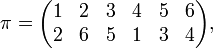$\pi = \begin{pmatrix} 1&2&3&4&5&6 \\ 2&6&5&1&3&4 \\ \end{pmatrix},$

so that two different permutations π connect τ with τ′ by a conjugacy relation.

The following summarizes the important result in the theory of the permutation group:

Two permutations are conjugate (belong to the same conjugacy class) if and only if they have the same cycle structure. A cycle structure (cycle shape) is defined uniquely by specifying the lengths λi of all cycles (including the 1-cycles). These lengths form a partition of N, i.e., a sequence {λ1, λ2, ..., λk} of positive integers such that$\lambda_1 \geq \lambda_2 \geq \cdots \geq \lambda_k \quad \hbox{and} \quad \sum_{i=1}^k \lambda_i = N.$

Example

The cycle structure of (1 4 3)(2 6) in S7 is given by λ1 = 3, λ2 = 2, λ3 = λ4 = 1 so that λ1 + λ2 + λ3+ λ4 = 7—to determine the cycle structure we must not forget the 1-cycles.

### Remark

According to elementary group representation theory, the number of conjugacy classes of a finite group equals the number of non-equivalent irreducible representations. Hence it is plausible that the irreducible representations of SN can be labeled by partitions of N. Graphically, partitions may be represented by Young diagrams named for the mathematician Alfred Young (1873 – 1940). Young developed an algorithm that indeed enables the construction of unique irreducible matrix representations of SN from Young diagrams (partitions of N).

## On the structure of the symmetric group

The group SN has proper normal subgroups if and only if N ≥ 3. For N > 4 the only proper normal subgroup of SN is the alternating group AN. When N = 4, there is an additional proper normal subgroup, often denoted V (isomorphic to Klein's Vierergruppe), consisting of the identity permutation and the permutations (1 2)(3 4), (1 3)(2 4), and (1 4)(2 3).

##  Orbits and Blocks

Let G be a subgroup of SN. The elements of G act also on {1,...,N}. One may define an equivalence relation, indicated by ~, on {1,...,N}, where i~j, i, j ∈ {1,...,N}, means that some element of G maps i to j. The resulting equivalence classes are called orbits. The groupG is called transitive if {1,...,N} forms one single orbit.

Let the orbits of G be O1 ... Ok. The action of G on Oi gives a homomorphism φi : G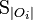$\text{S}_{|O_{i}|}$, where |Oi| is the order of Oi. While G is isomorphic to a subgroup of the product of the φi(G), this product is not, in general, isomorphic to G. For example, any G can be made to act on 2N points by using two copies of its action on N points. Yet no finite G, aside from the trivial group, is isomorphic to G×G.

While an intransitive permutation group may be broken up into orbits, a transitive permutation group has to be analyzed with greater subtlety: it could be primitive, or its action will be 'condensable' into an action on a set of blocks.

A primitive permutation group on the points {1,...,N} (it is assumed that N > 1 here) is, with only one exception, a group which leaves invariant no proper partition of {1,...,N}: A partition of {1,...,N} is an expression of {1,...,N} as a union of pairwise disjoint subsets. Any partition is proper, except for {1,...,N} = {1,...,N} and the expression of {1,...,N} as the union of its one-element subsets. Permutations of indices naturally induce permutations of partitions: for example, the permutation (1 2 3 4) sends the partition$\lbrace 1,2,3,4 \rbrace = \lbrace 1,2 \rbrace \cup \lbrace 3,4 \rbrace$ to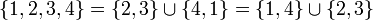$\lbrace 1,2,3,4 \rbrace = \lbrace 2,3 \rbrace \cup \lbrace 4,1 \rbrace = \lbrace 1,4 \rbrace \cup \lbrace 2,3 \rbrace$, sends the partition$\lbrace 1,2,3,4 \rbrace = \lbrace 2,3 \rbrace \cup \lbrace 1,4 \rbrace$ to the partition$\lbrace 1,2,3,4 \rbrace = \lbrace 3,4 \rbrace \cup \lbrace 1,2 \rbrace = \lbrace 1,2 \rbrace \cup \lbrace 3,4 \rbrace$, and sends the partition$\lbrace 1,2,3,4 \rbrace = \lbrace 1,3 \rbrace \cup \lbrace 2,4 \rbrace$ to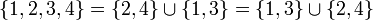$\lbrace 1,2,3,4 \rbrace = \lbrace 2,4 \rbrace \cup \lbrace 1,3 \rbrace = \lbrace 1,3 \rbrace \cup \lbrace 2,4 \rbrace$ (i.e., to itself). So the cycle (1 2 3 4) induces the permutation (12 34, 14 23)(13 24) on the partitions of {1,2,3,4} into 2 disjoint 2-element sets.

Since {1,2} has no proper partitions, the trivial subgroup of S2 would be considered primitive by the preceding, but it is not considered primitive: this is the sole exception referred to above. This is done for consistency: If N ≥ 3, then {1,...,N} has proper partitions. If G is trivial, then G fixes every partition of {1,...,N}, including the proper ones. If G is nontrivial but intransitive, the partition of {1,...,N} into orbits for the action of G will be proper (the nontriviality of G excludes the partition into singletons, while the intransitivity of G excludes the one-block partition), and it is a G-invariant partition of {1,...,N}. Thus primitive permutation groups are transitive, including the case when N=2.

## Note

1. Since some of the objects in the array may be identical and since the objects are ordered by their position in the array, it would be better to speak of a family—or a finite sequence—rather than of a set.